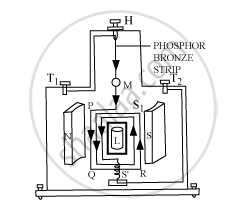# Draw a Labelled Diagram of a Moving Coil Galvanometer. Describe Briefly Its Principle and Working. - Physics

Draw a labelled diagram of a moving coil galvanometer. Describe briefly its principle and working.

#### Solution

Principle:

Its working is based on the fact that when a current carrying coil is placed in a magnetic field, it experiences a torque.Working:

Suppose the coil PQRS is suspended freely in the magnetic field.

Let l = length PQ or RS of the coil

b = breadth QR or SP of the coil

n = number of turns in the coil

Area of each turn of the coil, A = l × b

Let B = strength of the magnetic field in which the coil is suspended

I = current passing through the coil in the direction PQRS

At any instant, let α be the angle that the normal drawn on the plane of the coil makes with the direction of magnetic field.

The rectangular coil carrying current, when placed in the magnetic field, experiences a torque whose magnitude is given by:

τ = nIBA sinα

Due to the deflecting torque, the coil rotates and the suspension wire gets twisted. A restoring torque is set up in the suspension wire.

Let θ be the twist produced in the phosphor bronze strip due to the rotation of the coil and K be the restoring torque per unit twist of the phosphor bronze strip. Then, we have:

Total restoring torque produced = kθ

In equilibrium position of the coil, we have:

Deflecting torque = Restoring torque

NIBA = kθ

or

I=k/(NBA)theta " or "Gtheta

Here,

K/(NBA)=G=a["constant for a galvanometer"]

It is known as galvanometer constant.

Concept: Moving Coil Galvanometer
Is there an error in this question or solution?